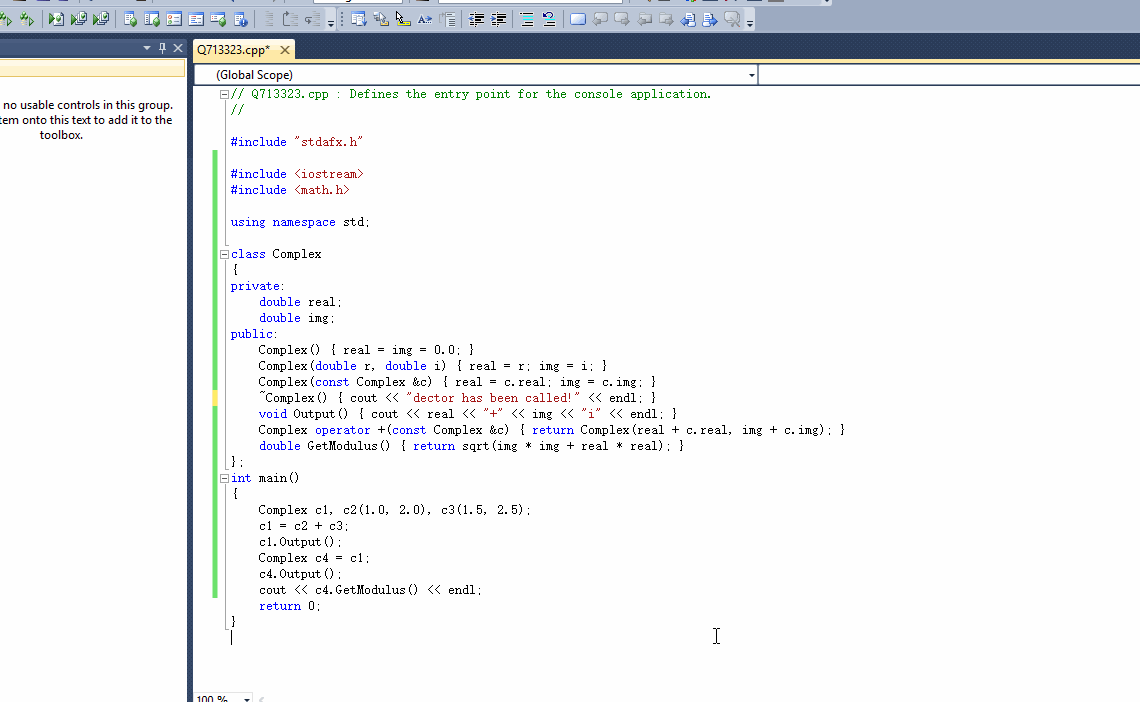C++类与对象 怎样利用复数类算复数的模等运算？

② 具有无参和有参的构造函数。
③ 具有深拷贝构造函数。
④ 具有析构函数。
⑤ 具有输出函数、求模函数、加法函数等。

2个回答

``````#include <iostream>
#include <math.h>

using namespace std;

class Complex
{
private:
double real;
double img;
public:
Complex() { real = img = 0.0; }
Complex(double r, double i) { real = r; img = i; }
Complex(const Complex &c) { real = c.real; img = c.img; }
~Complex() { cout << "dector has been called!" << endl; }
void Output() { cout << real << "+" << img << "i" << endl; }
Complex operator +(const Complex &c) { return Complex(real + c.real, img + c.img); }
double GetModulus() { return sqrt(img * img + real * real); }
};
int main()
{
Complex c1, c2(1.0, 2.0), c3(1.5, 2.5);
c1 = c2 + c3;
c1.Output();
Complex c4 = c1;
c4.Output();
cout << c4.GetModulus() << endl;
return 0;
}
```````如果问题得到解决，请点我回答左上角的采纳和向上的箭头`

C++中是有复数类的——complex.
C++标准程序库提供 complex 类，定义了复数对象。如果要用，必须在程序中包含这个头文件。#include.